# Percentage Worksheets

#### What Are Percentages?

Dealing with fractions can be difficult when you are performing mathematical operations in your daily lives. And percentages are another form of fraction; which causes havoc in the minds of the students. For instance, if you run a store, and you are telling the suppliers that 4/5th of your inventory is finished. He will most probably don’t understand you. Or you want to tell your customers that they get a 1/3rd discount on their entire purchase because of Christmas, they wouldn’t be using calculators at your store. Thus, comes the concept of percentage. It provides another way of representing factions and decimals. It just means per hundred. So, if you get 50%, that means 50 per hundred or half. A definite upgrade to the complicated decimal or fraction representation.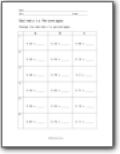###### Decimals to Percentages

Change the decimals to percentages.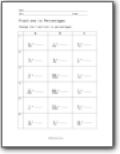###### Fractions to Percentages

Change the fractions to percentages.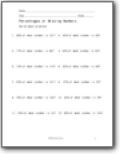###### Percentages of Missing Numbers

Solve each problem. Example: 98% of what number is 121?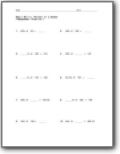###### Percent of a Number

Find round and decimal percentages of numbers.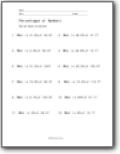###### Percentages of Numbers

You will be given a percentage of a number that you must convert.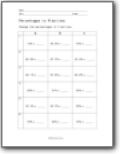###### Percentages to Fractions

Change the percentages to fractions.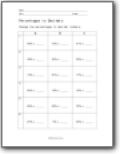###### Percentages to Decimals

Change the percentages to decimal numbers.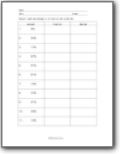###### Percentage Conversion

Convert each percentage to a fraction and a decimal.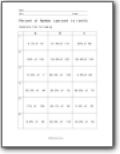###### Percent of Number (percent to tenth)

Complete the following types of problems: What is 43.6% of 125?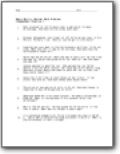###### Decimal Word Problems

Since we are working on percentages, these fit in nicely. Example: A car can run 11.45 km. per gallon of gas. If the tank holds 11.67 gallons of gas, how far (rounded off to second place of decimal) can the car run on one tank of gas?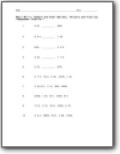###### Compare and Order Decimals, Percents and Fractions

You will learn how to compare all these different values.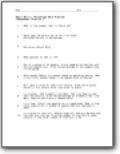###### Percentage Word Problems

We put all of the skills to use in story problems. Example problem: In a school, 384 students like Math, 232 like English and 184 like History. Find the percentage of students that like Math, English and History respectively?